We used R to analyze all examples in Chapter 13. We put the code here so that you can too.

Also see our lab on comparing two means using R.

• Data transformations:

marine$lnBiomassRatio <- log(marine$biomassRatio)
• Quantile plots:

qqnorm(marine$biomassRatio, datax = TRUE) • Mann-Whitney U-test (R uses the equivalent Wilcoxon rank-sum test): wilcox.test(timeToMating ~ feedingStatus, data = cannibalism) • Other new methods: • Shapiro-Wilk test of normality. • Sign test. • Permutation test. # Load required packages We’ll use the ggplot2 add on package to make graphs, and dplyr to calculate summary statistics by group. library(ggplot2) library(dplyr) # Example 13.1. Marine reserves The normal quantile plot, Shapiro-Wilk test of normality, and the log transformation, investigating the ratio of biomass between marine reserves and non-reserve control areas. ## Read and inspect data marine <- read.csv(url("https://whitlockschluter3e.zoology.ubc.ca/Data/chapter13/chap13e1MarineReserve.csv"), stringsAsFactors = FALSE) head(marine) ## biomassRatio ## 1 1.34 ## 2 1.96 ## 3 2.49 ## 4 1.27 ## 5 1.19 ## 6 1.15 ## Histogram A histogram visualizes the frequency distribution of biomass ratio (Figure 13.1-4). hist(marine$biomassRatio, right = FALSE, col = "firebrick",
las = 1, xlab = "Biomass ratio", main = "")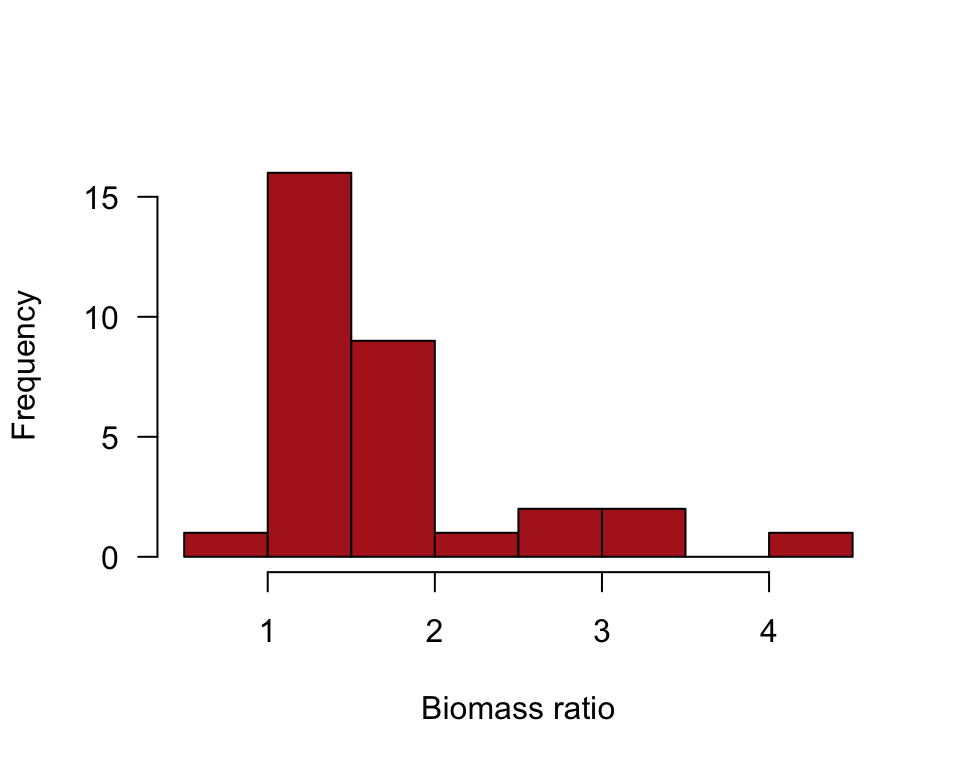or

ggplot(marine, aes(biomassRatio)) +
geom_histogram(fill = "firebrick", col = "black", binwidth = 0.5,
boundary = 0, closed = "left") +
labs(x = "Biomass ratio", y = "Frequency") +
theme_classic()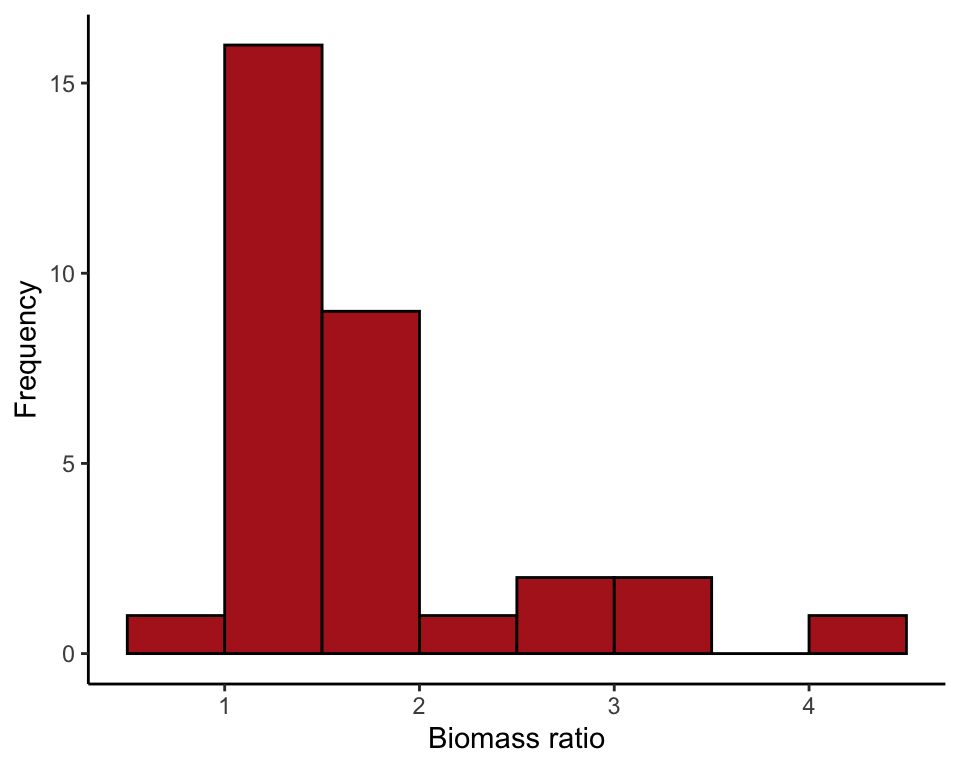## Normal quantile plot

The line in the normal quantile plot of Figure 13.1-4 passes through the first and 3rd quartiles of the data.

qqnorm(marine$biomassRatio, col = "firebrick", pch = 16, las = 1, datax = TRUE, xlab = "Biomass ratio") qqline(marine$biomassRatio, datax = TRUE, lwd = 2)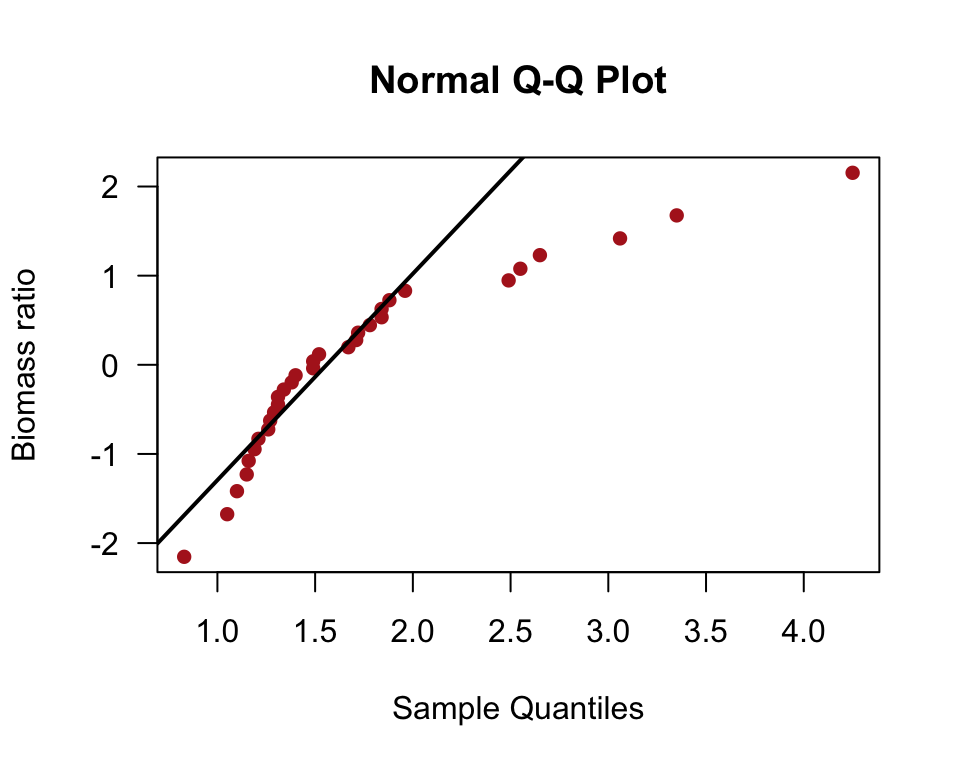## Shapiro-Wilk test

A test of the goodness of fit of the normal distribution to data.

shapiro.test(marine$biomassRatio) ## ## Shapiro-Wilk normality test ## ## data: marine$biomassRatio
## W = 0.81751, p-value = 8.851e-05

## Natural log-transformation

The function log() takes the natural logarithm of all the elements of a vector or variable. The following command saves the result as a new variable in the original data frame, marine.

marine$lnBiomassRatio <- log(marine$biomassRatio)

## Histogram

A histogram of the data after log-transformation of marine biomass ratio (Figure 13.3-2).

hist(marine$lnBiomassRatio, right = FALSE, col = "firebrick", las = 1, xlab = "Log biomass ratio", main = "", breaks = seq(-0.25, 1.5, by = 0.25))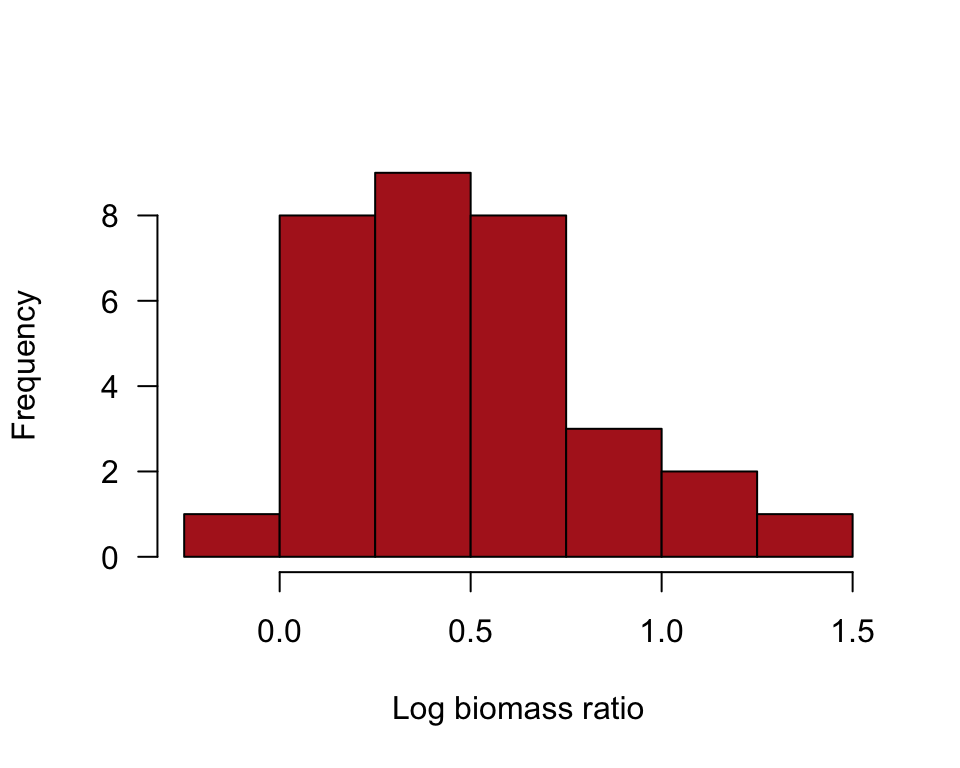or ggplot(marine, aes(lnBiomassRatio)) + geom_histogram(fill = "firebrick", col = "black", binwidth = 0.25, boundary = 0, closed = "left") + labs(x = "Log biomass ratio", y = "Frequency") + theme_classic()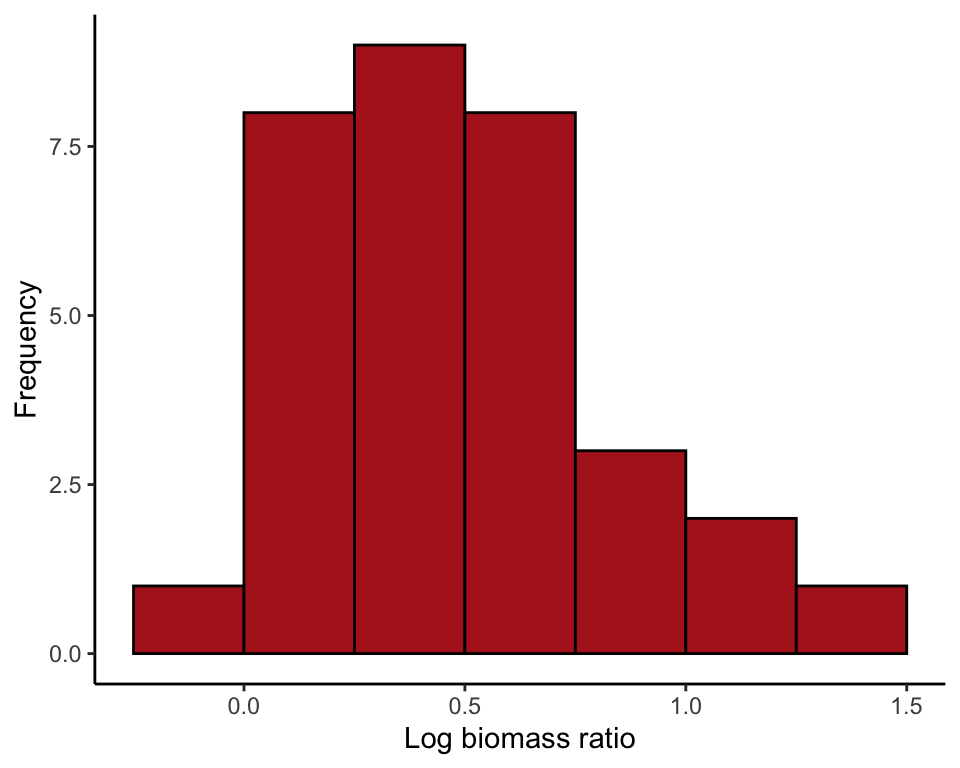## 95% CI of the mean Confidence interval for the mean of the log-transformed data. t.test(marine$lnBiomassRatio)$conf.int ##  0.3470180 0.6112365 ## attr(,"conf.level") ##  0.95 ## Back-transform 95% CI Transform the lower and upper limits of the confidence interval back to the original untransformed scale (exp() is the inverse of the natural log function). exp( t.test(marine$lnBiomassRatio)$conf.int ) ##  1.414842 1.842708 ## attr(,"conf.level") ##  0.95 # Example 13.4. Sexual conflict The sign test, comparing the numbers of species in 25 pairs of closely related insect taxa. ## Read and inspect data. conflict <- read.csv(url("https://whitlockschluter3e.zoology.ubc.ca/Data/chapter13/chap13e4SexualConflict.csv"), stringsAsFactors = FALSE) head(conflict) ## taxonPair nSpeciesMultipleMating nSpeciesSingleMating difference ## 1 A 53 10 43 ## 2 B 73 120 -47 ## 3 C 228 74 154 ## 4 D 353 289 64 ## 5 E 157 30 127 ## 6 F 300 4 296 ## Histogram A histogram of the difference in numbers of species (Figure 13.4-1). hist(conflict$difference, right = FALSE, col = "firebrick",
breaks = 50, xlab = "Difference in species number", las = 1)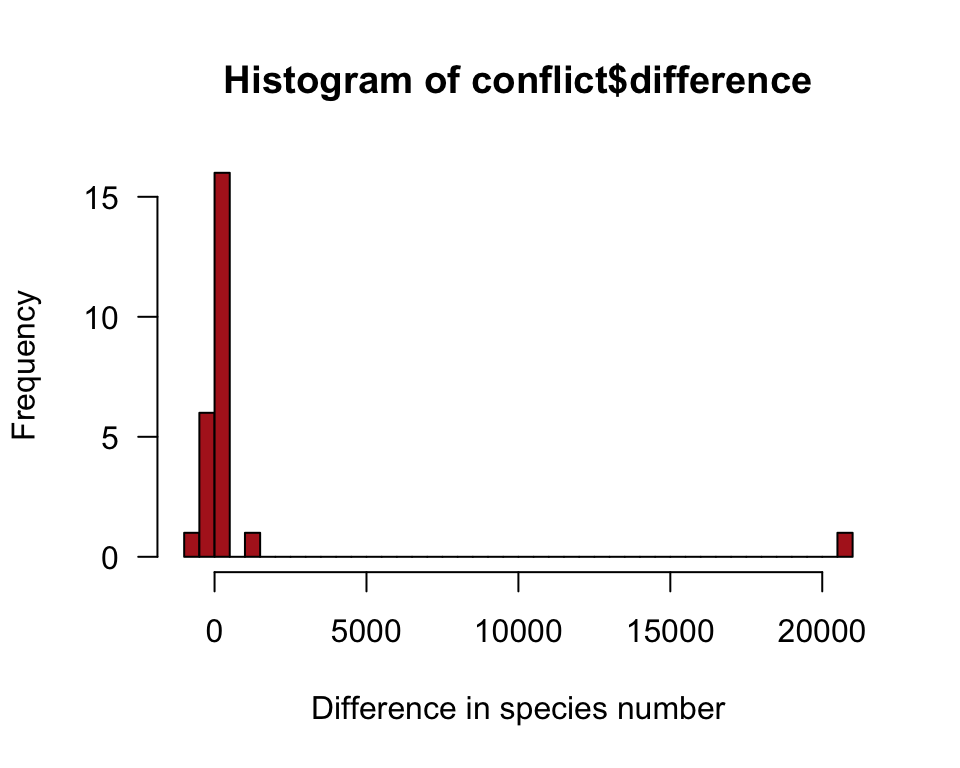## Frequencies

Count up the frequency of differences that are below, equal to, and above zero.

The command below creates a new variable in the data frame called conflictZero to indicate whether each difference is below, equal to, or above 0.

conflictZero <- cut(conflict$difference, breaks = c(-Inf, 0, 1, Inf), labels = c("below","equal","above"), right = FALSE) table(conflictZero) ## conflictZero ## below equal above ## 7 0 18 ## Sign test The sign test is just a binomial test. The output includes a confidence interval for the proportion using the Clopper-Pearson method, which isn’t covered in the book. binom.test(7, n = 25, p = 0.5) ## ## Exact binomial test ## ## data: 7 and 25 ## number of successes = 7, number of trials = 25, p-value = 0.04329 ## alternative hypothesis: true probability of success is not equal to 0.5 ## 95 percent confidence interval: ## 0.1207167 0.4938768 ## sample estimates: ## probability of success ## 0.28 # Example 13.5. Cricket cannibalism **The Wilcoxon rank-sum test (equivalent to the Mann-Whitney U*-test) comparing times to mating (in hours) of starved and fed female sagebrush crickets. We also apply the permutation test** to the same data*. ## Read and inspect data cannibalism <- read.csv(url("https://whitlockschluter3e.zoology.ubc.ca/Data/chapter13/chap13e5SagebrushCrickets.csv"), stringsAsFactors = FALSE) head(cannibalism) ## feedingStatus timeToMating ## 1 starved 1.9 ## 2 starved 2.1 ## 3 starved 3.8 ## 4 starved 9.0 ## 5 starved 9.6 ## 6 starved 13.0 ## Multiple histograms The following commands produce Figure 13.5-1. We begin by ordering the categories of the feeding status variable so that “starved” comes before “fed” in the histogram. cannibalism$feedingStatus <- factor(cannibalism$feedingStatus, levels = c("starved", "fed")) ggplot(cannibalism, aes(x = timeToMating)) + geom_histogram(fill = "firebrick", col = "black", binwidth = 20, boundary = 0, closed = "left") + facet_wrap( ~ feedingStatus, ncol = 1, scales = "free_y") + labs(x = "Time to mating (hours)", y = "Frequency") + theme_classic()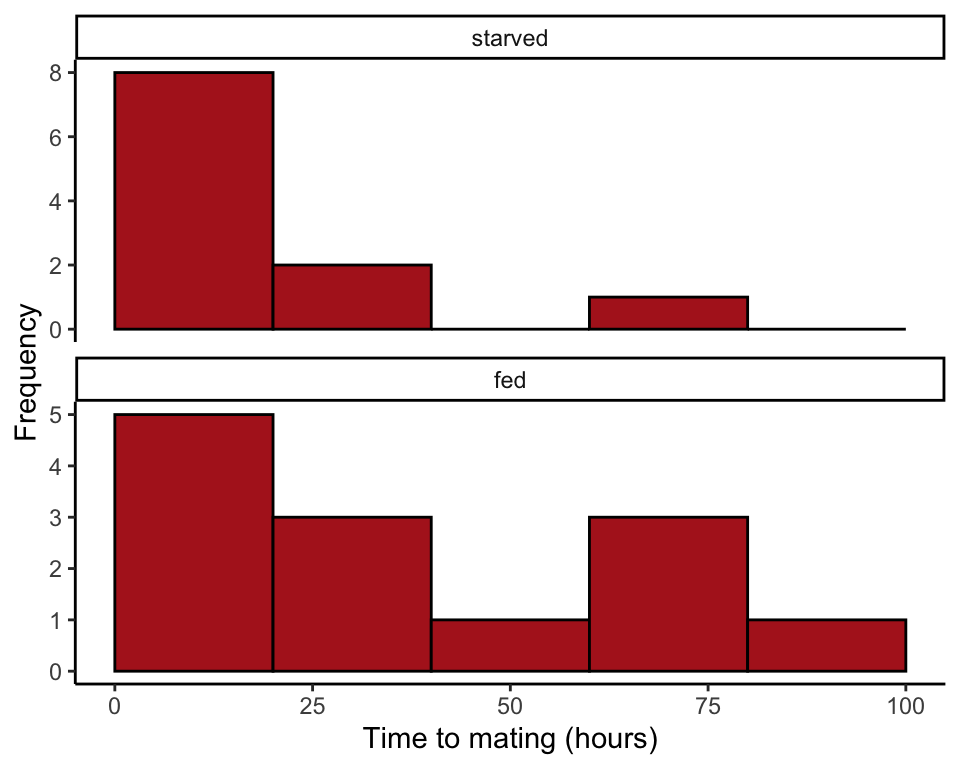## Wilcoxon rank-sum test This test, available in R, is equivalent to the Mann-Whitney U-test. wilcox.test(timeToMating ~ feedingStatus, data = cannibalism) ## ## Wilcoxon rank sum test ## ## data: timeToMating by feedingStatus ## W = 55, p-value = 0.3607 ## alternative hypothesis: true location shift is not equal to 0 # Permutation test Permutation test of the difference between mean time to mating of starved and fed crickets. ## Observed value Begin by calculating the observed difference between means (starved minus fed). The difference is -18.25734 in this data set. cricketMeans <- summarize(group_by(cannibalism, feedingStatus), timeToMating = mean(timeToMating, na.rm = TRUE)) cricketMeans <- as.data.frame(cricketMeans) cricketMeans ## feedingStatus timeToMating ## 1 starved 17.72727 ## 2 fed 35.98462 diffMeans <- cricketMeans$timeToMating - cricketMeans$timeToMating diffMeans ##  -18.25734 ## Number of permutations Decide on the number of permutations. We use 10K here. nPerm <- 10000 ## Run a loop Create a loop to permute the data many times (the number of times determined by nperm). In the following loop, i is a counter that climbs from 1 to nPerm by 1. In each iteration, the permuted difference is saved in the object permResult. permResult <- vector() # initializes for(i in 1:nPerm){ # step 1: permute the times to mating cannibalism$permSample <- sample(cannibalism$timeToMating, replace = FALSE) # step 2: calculate difference betweeen means permSampleMean <- as.data.frame(summarize(group_by(cannibalism, feedingStatus), permMean = mean(permSample, na.rm = TRUE))) permResult[i] <- permSampleMean$permMean - permSampleMean\$permMean
}

## Plot null distribution

Make a histogram of the permuted differences (Figure 13.8-1).

hist(permResult, right = FALSE, breaks = 100)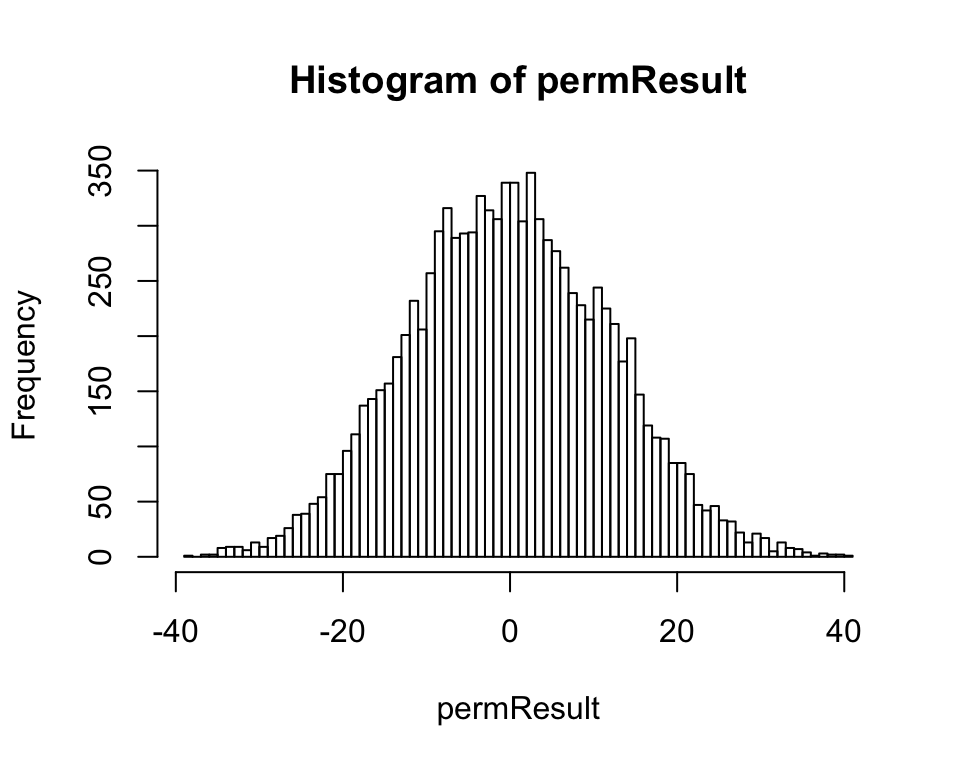or

ggplot(data.frame(permResult), aes(permResult)) +
geom_histogram(fill = "white", col = "black", binwidth = 1,
boundary = 0, closed = "left") +
labs(x = "Permuted difference in treatment means (hours)", y = "Frequency") +
theme_classic()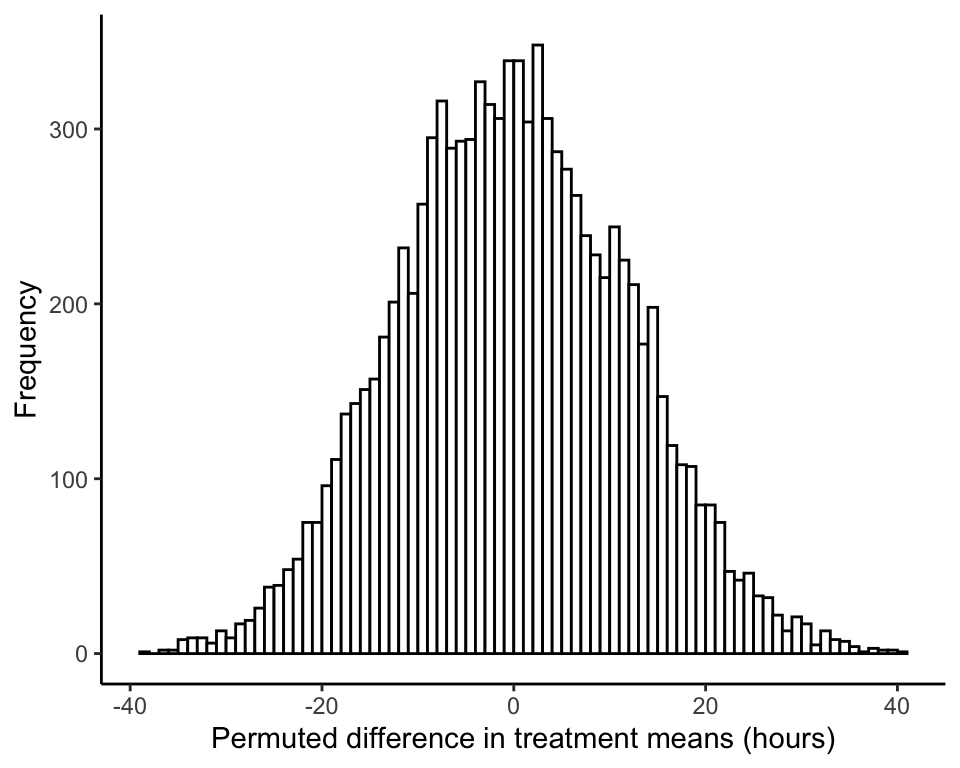## Approximate $$P$$-value

Use the null distribution to calculate an approximate P-value. This is the twice the proportion of the permuted means that fall below the observed difference in means, diffMeans (-18.25734 in this example). The following code calculates the number of permuted means falling below diffMeans.

sum(as.numeric(permResult <= diffMeans))
##  649

These commands obtain the fraction of permuted means falling below diffMeans.

sum(as.numeric(permResult <= diffMeans)) / nPerm
##  0.0649

Finally, multiply by 2 to get the P-value for a two-sided test. The value won’t be identical to the one in the book, or to the value you obtain, because 10,000 iterations is not large enough for extreme accuracy.

2 * ( sum(as.numeric(permResult <= diffMeans)) / nPerm )
##  0.1298